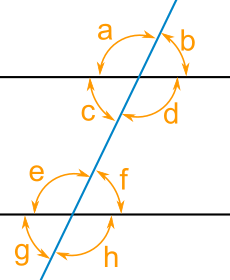• ­­­­Ramapo High School                               Physics                                      Dr. Lu

Review question for measurement

The diameter of an automobile tire is closest to   (1) 10 - 2   m    (2)  100 m    (3) 101 m    (4) 102 m    (#36-2015)

Geometry

1.Areas

• TriangleTriangle area  = ½ (base)(height)

• Trapezoid ATrapezoid Area =  (1/2) (height)(b1 + b2 )  = (1/2)(height)(a+b)

1. Parallel linesQuestions

Vertical angles are :

Corresponding angles are:

Alternate interior angles are:

Alternate exterior angles :

Consecutive interior angles are :

3.How to draw parallel lines

Method 1: Video

Method 2: Or by using a triangular scale and straight edge (or scale ri=uler)

Example: How to draw parallel lines to line P and line Q respectively in the following

to form a parallelogram?Algebra 1: Graphical Method

Linear function y = mx +b

-  Graphing from points

- What is representing the slope in  y=mx b

- How to calculate the slope?

- What is the meaning of sloe?

[Note]

function f(x) = y = mx + b

Where x is the independent variable, y is the dependent variable, m  is the slope and b is

the y-intercept.where slope = m =3 and the y intercept =2

Example 1

Graph the linear equation y = 2x +1

(1) Data table

x        y =mx +b =2x +1

-1        y =2x+ 1= 2(-1) +1 = -1

0        y= 2(0) +1 =

1        y = 2(1) +1 =3

(2) Find the points ( -1, -1), (0, 1), and (1,3)

(3) Then graph it

- Draw X and Y axis

- Scale on the X and Y axis

- Label the quantity for x-axis  and for y-axis

- Graph the points

- Connecting the points

- Draw a straight line

- Label the straight line as  y = 2x + 1

This graph for Physics problem is similar to the way as shown above.

Check for understanding, group work, individual work, summary, reflection, and exit ticket will be in the attached file. https://classroom.google.com/w/MTc3MTIxNzA4Mjda/t/all